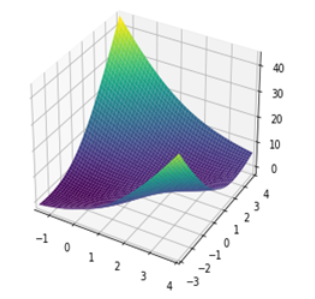top of page
Search

# Python implementation of McCormick Function

Updated: Aug 5, 2021Mathematical DefinitionInput Domain

The input range of the func is: x1∈ [-1.5, 4], x2∈ [-3, 4].

Global Minima

The func has one global min f(x*)=-1.9133, at x* = (0.54719,-1.54719)

Characteristics

The function is continuous.

The function is  convex.

The function is defined on 2-D space.

The function is multimodal.

The function is differentiable.

Python Implementation

```% Please forward any comments or bug reports in chat
Copyright 2021. INDUSMIC PRIVATE LIMITED.THERE IS NO WARRANTY, EXPRESS OR IMPLIED. WE DO NOT ASSUME ANY LIABILITY FOR THE USE OF THIS PROGRAM. If software is modified to produce derivative works, such modified software should be clearly marked. Additionally, user can redistribute it and/or modify it under the terms of the GNU General Public License. This program is distributed in the hope that it will be useful, but WITHOUT ANY WARRANTY. See the GNU General Public License for more details.
% for any support connect with us on help.indusmic@gmail.com
# Author: Dhanishtha Sharma

import sympy
import math
from sympy import symbols
from sympy import *
from sympy.plotting import plot3d
import numpy as np
import matplotlib as mpl
import matplotlib.pyplot as plt
from mpl_toolkits.mplot3d import Axes3D

%matplotlib notebook
plt.rcParams['figure.figsize'] = (6,4)
plt.rcParams['figure.dpi']=150
fig=plt.figure()
x1,x2=symbols('x1 x2')
plot3d((sin(x1+x2)+(x1-x2)**2-1.5*x1+2.5*x2+1),(x1,-1.5,4,2),(x2,-3,4,2))

```

References:

 Jamil, Momin, and Xin-She Yang. "A literature survey of benchmark functions for global optimization problems." International Journal of Mathematical Modelling and Numerical Optimization 4.2 (2013): 150-194.